SAS Examples: Proc Freq

# SAS Examples: Proc Freq

Last updated:

## Sample data

data mytable;

input id= name=$12. balance= state=$2.;

DATALINES;
id=1 name=alice balance=5.12 state=NE
id=2 name=bob  balance=12.31 state=SC
id=3 name=charlie balance=45.14 state=NY
id=4 name=daniel balance=32.78 state=
id=5 name=alice balance=20.23 state=WA
id=6 name=bob balance=70.89 state=NM
id=7 name=gabe balance=29.67 state=NM
id=8 name=charlie balance=55.66 state=CA
id=9 name=ivan balance= state=
;
run;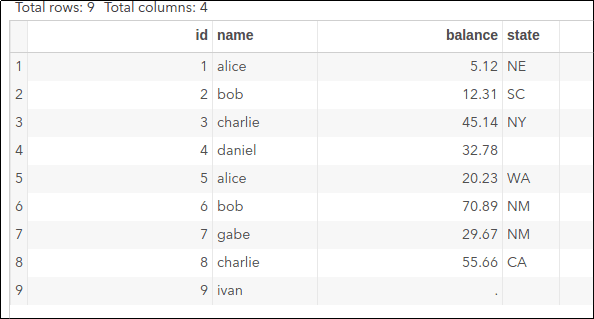Original data

## Simplest possible proc freq

Outputs the frequency for each value in column <column> on table <library>.<source_table>.

proc freq data=mytable;
tables state;
run;original dataSimple proc freq output for the state column

## Save output to another table

Do proc freq while also saving the results to othertable

proc freq data=mytable;
tables <column> / out=othertable;
run;


## Show missing data separately

Output a frequency table and a histogram for column <column> on table <library>.<source_table>, including missing data as a separate category.

proc freq data=mytable;
tables state / missing;
run;original data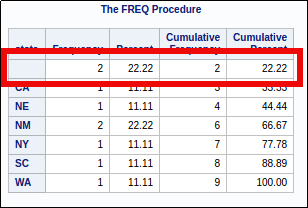The marked row shows stats for missing values

## Proc freq with histogram

Output a frequency table and a histogram for column state.

ods graphics on;
proc freq data=mytable;
tables state / plots=freqplot();
run;original data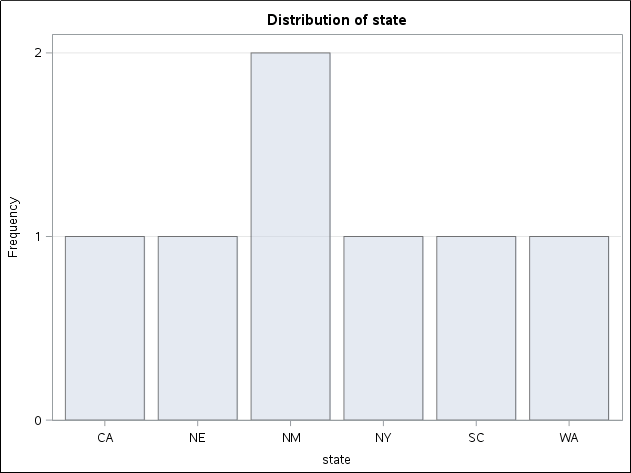Note that missing data are not included.

## Histogram with continuous data

It's very common to create a FORMAT to bin your data into separate groups, so that you can view continuous data as buckets.

proc format;
value balance_bins
0       = "Exactly 0"
0 -< 10 = "Up to US$10" 10 -< 20 = "From US$ 10 to US$20" 20 -< 30 = "From US$ 20 to US$30" 30 -< 40 = "From US$ 30 to US$40" 40 -< 50 = "From US$ 40 to US$50" 50 - high = "Above US$ 50"
. = "No data"
other = "???";
run;

ods graphics on;
proc freq data=mytable;
tables balance/ missing plots=freqplot;
format balance balance_bins.;
run;original data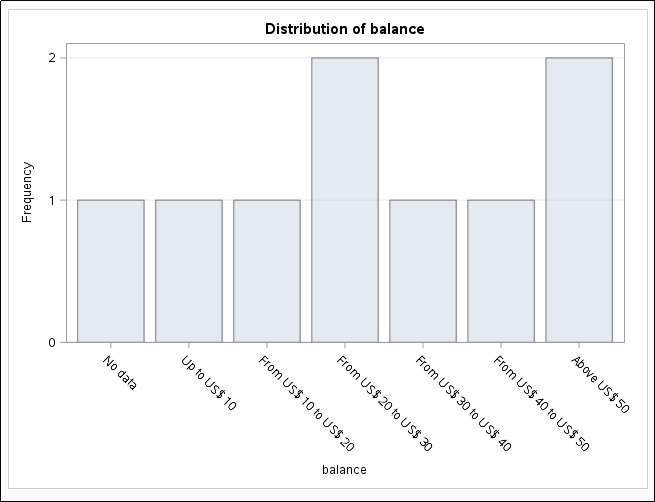Use can use formats to group continuous variables into buckets

## Two-way histogram

You want to see the distribution of one categorical variable versus the other.

This will group the results by the FIRST element, namely state. You can also use groupby=column to group the results by name

ods graphics on;
proc freq data=mytable;
tables state*name / plots=freqplot(groupby=row twoway=stacked);
run;original data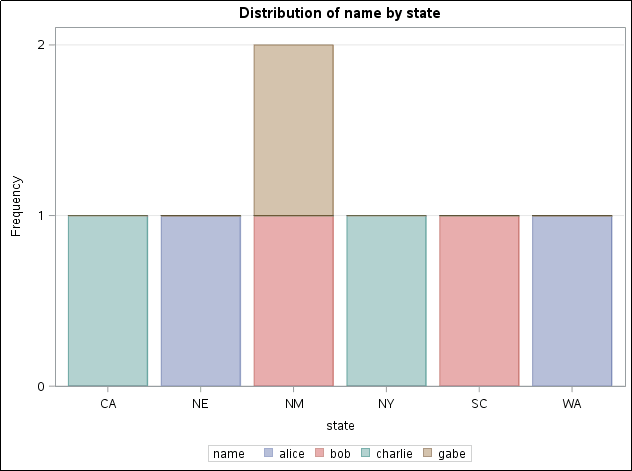Two-way proc freq histogram

## Two-way histogram with continuous data

Again, use proc format to turn continuous into discrete data:

proc format;
value balance_bins
0       = "Exactly 0"
0 -< 10 = "Up to US$10" 10 -< 20 = "From US$ 10 to US$20" 20 -< 30 = "From US$ 20 to US$30" 30 -< 40 = "From US$ 30 to US$40" 40 -< 50 = "From US$ 40 to US$50" 50 - high = "Above US$ 50"
. = "No data"
other = "???";
run;

ods graphics on;
proc freq data=mytable;
tables state*balance / plots=freqplot(groupby=row twoway=stacked);
format balance balance_bins.;
run;original data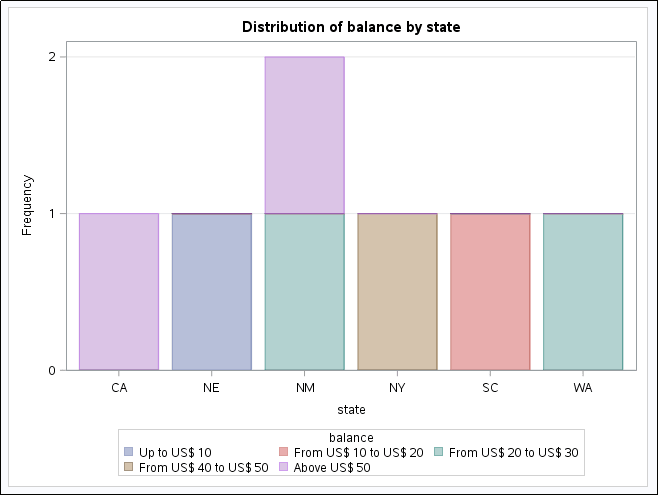You can also use formats for two-way plots